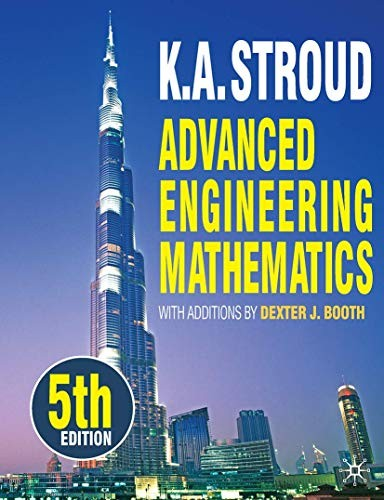# Advanced Engineering Mathematics by K. A. Stroud

In Stock
\$57.99
+10% GST

A long-standing, best-selling, comprehensive textbook covering all the mathematics required on upper level engineering mathematics undergraduate courses.

Only 1 left

### Advanced Engineering Mathematics by K. A. Stroud

A long-standing, best-selling, comprehensive textbook covering all the mathematics required on upper level engineering mathematics undergraduate courses. Its unique programmed approach takes students through the mathematics they need in a step-by-step fashion with a wealth of examples and exercises. The text demands that students engage with it by asking them to complete steps that they should be able to manage from previous examples or knowledge they have acquired, while carefully introducing new steps. By working with the authors through the examples, students become proficient as they go. By the time they come to trying examples on their own, confidence is high.

This textbook is ideal for undergraduates on upper level courses in all Engineering disciplines and Science.

### Why buy from World of BooksOur excellent value books literally don't cost the earthFree delivery in AustraliaEvery used book bought is one saved from landfill

'[E]ntirely fit for purpose.' - Times Higher Education Supplement 'Stroud is by far and away the best text book for engineering maths. It is excellent for students to use on their own to reinforce what they have seen in class.' - Esther Norton, Anglia Ruskin University, UK 'This is quite simply the BEST text in the area' - Justin Whitty, University of Central Lancashire, UK 'I recommend this book for self study by students due to the "step by step" approach.' - Alan Crocker, University of the West of England, UK Current reviews of the previous edition from amazon.co.uk: 'This book is essential for undergraduates studying an engineering degree course. Stroud's books fill a vast deficit found in most higher level maths text books, i.e. his books fill in procedural gaps other text books assume will be filled in while attending lectures. Stroud does not 'assume', he teaches...' 'This book is extremely useful...[It] has well written and easy to follow chapters on many different areas. To back this up it has useful examples for each section building up from simple cases to more complex exam style questions. This book really is excellent. If you get one maths book for engineering, get this one.' 'To anyone who is about to undertake, or is indeed in the middle of any engineering degree, this book is a must. The layout and style are cleverly designed to keep you working through the chapters. Don't do engineering without a copy.'

K.A. Stroud Formerly Principal Lecturer in the Department of Mathematics at Lanchester Polytechnic (now Coventry University), UK. He is also the author of Foundation Mathematics and Engineering Mathematics, companion volumes to this book.

Dexter J. Booth Formerly Principal Lecturer in the School of Computing and Engineering at the University of Huddersfield, UK. He is the author of several mathematics textbooks and is co-author of Foundation Mathematics and the sixth edition of Engineering Mathematics.

Hints on Using the Book.- Useful Background Information.- Numerical Solutions of Equations and Interpolation.- Laplace Transforms Part 1.- Laplace Transforms Part 2.- Laplace Transforms Part 3.- Difference equations and the Z Transform.- Introduction to invariant linear systems.- Fourier Series 1 .- Fourier Series 2.- Introduction to the Fourier Transform.- Power Series Solutions of Ordinary Differential Equations 1.- Power Series Solutions of Ordinary Differential Equations 2.- Power Series Solutions of Ordinary Differential Equations 3.- Numerical Solutions of Ordinary Differential Equations.- Partial Differentiation.- Partial Differential Equations.- Matrix Algebra .- Systems of ordinary differential equations.- Numerical Solutions of Partial Differential Equations.- Multiple Integration Part 1.- Multiple Integration Part 2.- Integral Functions.- Vector Analysis Part 1.- Vector Analysis Part 2.- Vector Analysis Part 3.- Complex Analysis Part 1.- Complex Analysis Part 2.- Complex Analysis Part 3.- Optimization and Linear Programming.

GOR007640479
Advanced Engineering Mathematics by K. A. Stroud
K. A. Stroud